Confidence estimation

(diff) ← Older revision | Latest revision (diff) | Newer revision → (diff)

A method in mathematical statistics for the construction of a set of approximate values of the unknown parameters of probability distributions.

Letbe a random vector assuming values in a setin a Euclidean space and let the probability distribution of this vector belong to the parametric family of distributions defined by the densities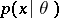,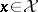,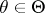, with respect to some measure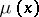. It is assumed that the true value of the parametric pointcorresponding to the result of observations ofis unknown. Confidence estimation consists in constructing a certain set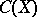, depending on, containing the value of a given function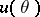corresponding to the unknown true value of.

Letbe the range of values of the function,, and let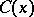,, be some family of sets belonging tofor allfrom; moreover, it is assumed that for an arbitrary elementand any value ofthe probability of the eventis defined. This probability is given by the integraland is said to be the covering probability of the value ofby the setfor a given value of.

If the true value ofis unknown, the set(from the family of sets,) which corresponds to the results of observations ofis said to be a confidence set or an interval estimator for the unknown true value of the function. The confidence probability, which can be expressed, in terms of the covering probability, by the equation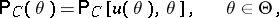is employed as the probability characteristic of the interval estimatorconstructed according to the above rule. In other words,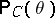is the probability of covering by the setof the value of a given functioncorresponding to the unknown true parametric point.

If the confidence probabilityis independent of, the interval estimatoris said to be similar to the sampling space. This name is due to the analogy between the formulas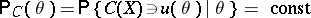and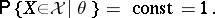In a more general situationdepends on the unknown, and for this reason the quality of the interval estimator is usually characterized in practical work by the confidence level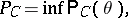where the lower bound is calculated over the set. (The confidence level is sometimes called the confidence coefficient.)

Optimization of confidence estimation is defined by the requirements to be met by interval estimators. For instance, if the objective is to construct confidence sets similar to sampling spaces, with a given confidence level(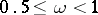), the first requirement is expressed by the identityIt is natural to look for an interval estimator that covers the true value ofwith a probability at least that of covering an arbitrary value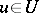. In other words, the second requirement, known as the requirement of unbiasedness, is expressed by the inequality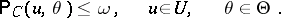Under these conditions, the "best" interval estimatormay reasonably be taken as one which covers any valueother than the true valuewith a smaller probability. Hence the third requirement of "highest accuracy" : For any set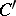other thanand meeting the condition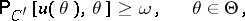the inequality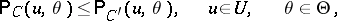must be valid.

The task of finding interval estimatorssatisfying all three requirements is equivalent to the task of constructing unbiased most-powerful statistical tests similar to the sampling space and having significance level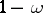. The problem of the existence of a solution to this problem and its constructive description form the base of the general theory of statistical hypothesis testing.

Confidence estimation is most often used whenis a scalar function. Let,, be independent random variables subject to the same normal distribution, with the unknown parametersand. The problem is to construct an interval estimator for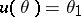. Let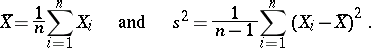Since the random variable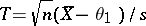is subject to the Student distribution with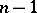degrees of freedom, and since this distribution does not depend on the unknown parameters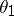and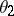(,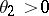), it follows that, for any positive, the probability of occurrence of the eventdepends only on. If this interval is taken as the interval estimatorfor, it will correspond to the confidence probabilitywhich is independent of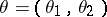. Such an interval estimator is known as a confidence interval, while its end points are known as confidence bounds; in this case the confidence interval is a confidence estimator similar to the sampling space. In this example the interval estimator is most accurate unbiased.

How to Cite This Entry:
Confidence estimation. Encyclopedia of Mathematics. URL: http://encyclopediaofmath.org/index.php?title=Confidence_estimation&oldid=12600
This article was adapted from an original article by Yu.V. LinnikN.M. Khalfina (originator), which appeared in Encyclopedia of Mathematics - ISBN 1402006098. See original article